Publicité

# S3 Chapter 2 Fluid Pressure

27 Jul 2013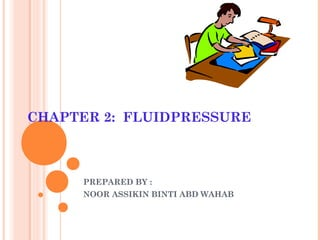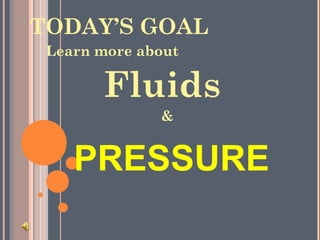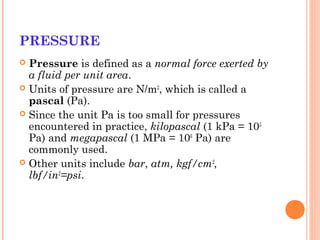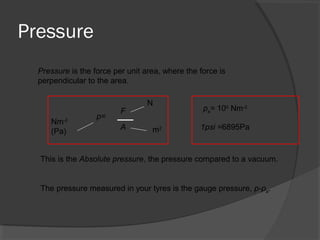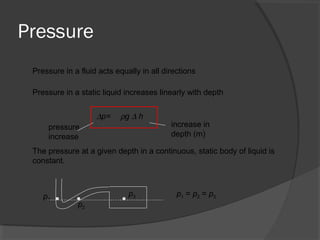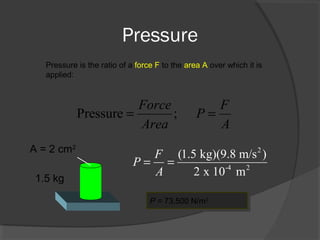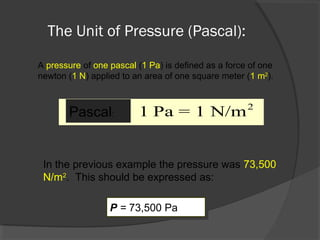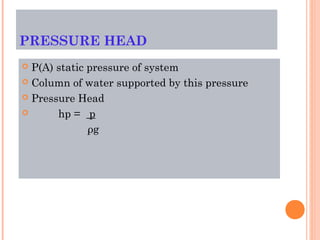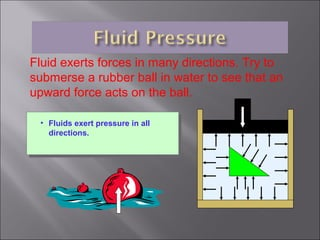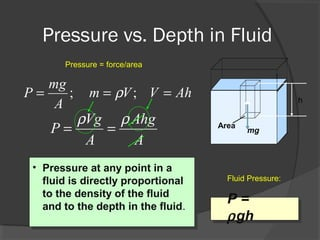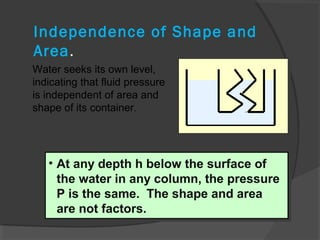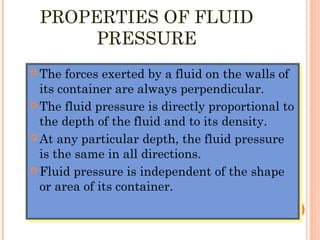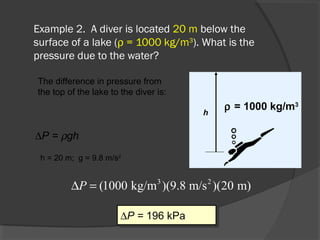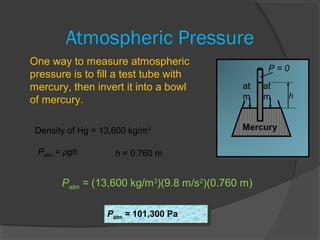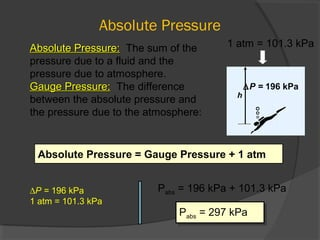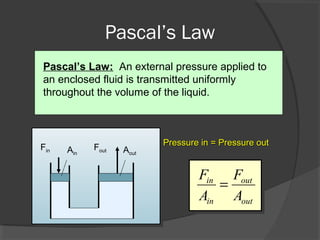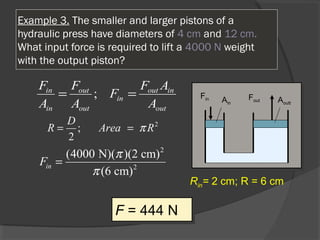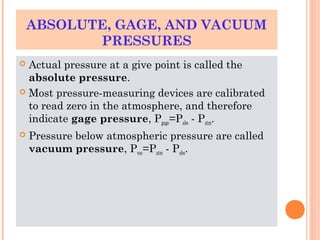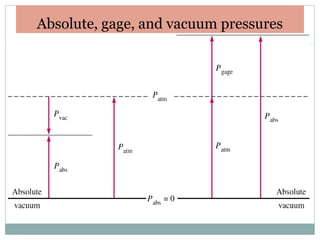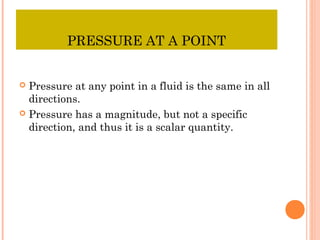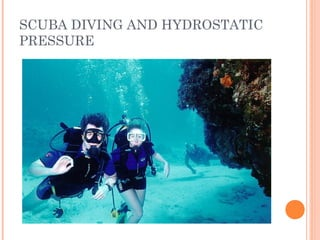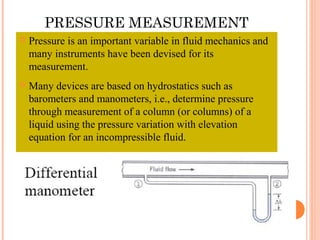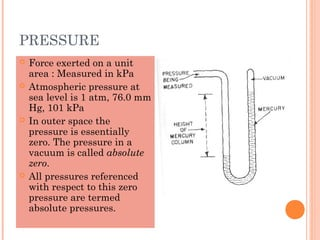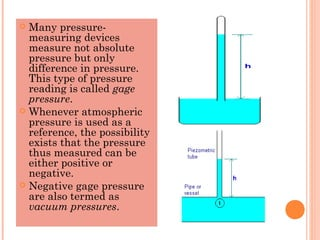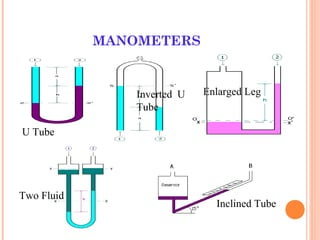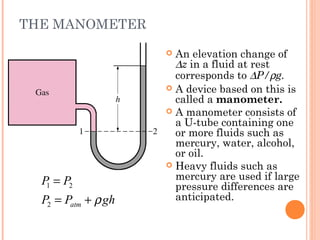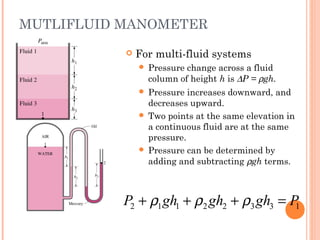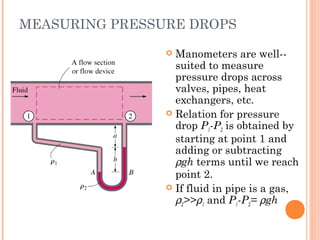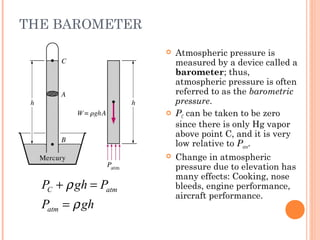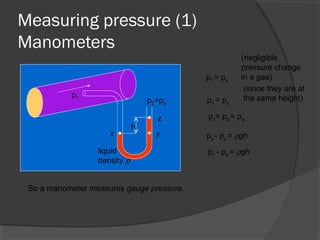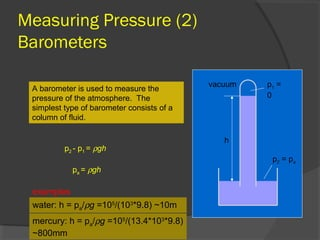1 sur 31
Publicité

### S3 Chapter 2 Fluid Pressure

1. CHAPTER 2: FLUIDPRESSURE PREPARED BY : NOOR ASSIKIN BINTI ABD WAHAB
3. PRESSURE  Pressure is defined as a normal force exerted by a fluid per unit area.  Units of pressure are N/m2 , which is called a pascal (Pa).  Since the unit Pa is too small for pressures encountered in practice, kilopascal (1 kPa = 103 Pa) and megapascal (1 MPa = 106 Pa) are commonly used.  Other units include bar, atm, kgf/cm2 , lbf/in2 =psi.
4. Pressure Pressure is the force per unit area, where the force is perpendicular to the area. p= A m2 Nm-2 (Pa) N F This is the Absolute pressure, the pressure compared to a vacuum. pa= 105 Nm-2 1psi =6895Pa The pressure measured in your tyres is the gauge pressure, p-pa.
5. Pressure Pressure in a fluid acts equally in all directions Pressure in a static liquid increases linearly with depth ∆p= increase in depth (m) pressure increase ρg ∆ h The pressure at a given depth in a continuous, static body of liquid is constant. p1 p2 p3 p1 = p2 = p3
6. Pressure Pressure is the ratio of a force F to the area A over which it is applied: Pressure ; Force F P Area A = = A = 2 cm2 1.5 kg 2 -4 2 (1.5 kg)(9.8 m/s ) 2 x 10 m F P A = = P = 73,500 N/m2P = 73,500 N/m2
7. The Unit of Pressure (Pascal): A pressure of one pascal (1 Pa) is defined as a force of one newton (1 N) applied to an area of one square meter (1 m2 ). 2 1 Pa = 1 N/mPascal: In the previous example the pressure was 73,500 N/m2 . This should be expressed as: P = 73,500 PaP = 73,500 Pa
8. PRESSURE HEAD  P(A) static pressure of system  Column of water supported by this pressure  Pressure Head  hp = p ρg
9. Fluid exerts forces in many directions. Try to submerse a rubber ball in water to see that an upward force acts on the ball. • Fluids exert pressure in all directions. F
10. Pressure vs. Depth in Fluid Pressure = force/area ; ; mg P m V V Ah A ρ= = = Vg Ahg P A A ρ ρ = = h mg Area • Pressure at any point in a fluid is directly proportional to the density of the fluid and to the depth in the fluid. P = ρgh Fluid Pressure:
11. Independence of Shape and Area. Water seeks its own level, indicating that fluid pressure is independent of area and shape of its container. • At any depth h below the surface of the water in any column, the pressure P is the same. The shape and area are not factors. • At any depth h below the surface of the water in any column, the pressure P is the same. The shape and area are not factors.
12. PROPERTIES OF FLUID PRESSURE  The forces exerted by a fluid on the walls of its container are always perpendicular.  The fluid pressure is directly proportional to the depth of the fluid and to its density.  At any particular depth, the fluid pressure is the same in all directions.  Fluid pressure is independent of the shape or area of its container.  The forces exerted by a fluid on the walls of its container are always perpendicular.  The fluid pressure is directly proportional to the depth of the fluid and to its density.  At any particular depth, the fluid pressure is the same in all directions.  Fluid pressure is independent of the shape or area of its container.
13. Example 2. A diver is located 20 m below the surface of a lake (ρ = 1000 kg/m3 ). What is the pressure due to the water? h ρ = 1000 kg/m3 ∆P = ρgh The difference in pressure from the top of the lake to the diver is: h = 20 m; g = 9.8 m/s2 3 2 (1000 kg/m )(9.8 m/s )(20 m)P∆ = ∆P = 196 kPa∆P = 196 kPa
14. Atmospheric Pressure at m at m h Mercury P = 0 One way to measure atmospheric pressure is to fill a test tube with mercury, then invert it into a bowl of mercury. Density of Hg = 13,600 kg/m3 Patm = ρgh h = 0.760 m Patm = (13,600 kg/m3 )(9.8 m/s2 )(0.760 m) Patm = 101,300 PaPatm = 101,300 Pa
15. Absolute Pressure Absolute Pressure:Absolute Pressure: The sum of the pressure due to a fluid and the pressure due to atmosphere. Gauge Pressure:Gauge Pressure: The difference between the absolute pressure and the pressure due to the atmosphere: Absolute Pressure = Gauge Pressure + 1 atmAbsolute Pressure = Gauge Pressure + 1 atm h ∆P = 196 kPa 1 atm = 101.3 kPa ∆P = 196 kPa 1 atm = 101.3 kPa Pabs = 196 kPa + 101.3 kPa Pabs = 297 kPaPabs = 297 kPa
16. Pascal’s Law Pascal’s Law: An external pressure applied to an enclosed fluid is transmitted uniformly throughout the volume of the liquid. FoutFin AoutAin Pressure in = Pressure outPressure in = Pressure out in out in out F F A A =
17. Example 3. The smaller and larger pistons of a hydraulic press have diameters of 4 cm and 12 cm. What input force is required to lift a 4000 N weight with the output piston? Fout Fin AouttAin ;in out out in in in out out F F F A F A A A = = 2 2 (4000 N)( )(2 cm) (6 cm) inF π π = 2 ; 2 D R Area Rπ= = F = 444 NF = 444 N Rin= 2 cm; R = 6 cm
18. ABSOLUTE, GAGE, AND VACUUM PRESSURES  Actual pressure at a give point is called the absolute pressure.  Most pressure-measuring devices are calibrated to read zero in the atmosphere, and therefore indicate gage pressure, Pgage=Pabs - Patm.  Pressure below atmospheric pressure are called vacuum pressure, Pvac=Patm - Pabs.
19. Absolute, gage, and vacuum pressures
20. PRESSURE AT A POINT  Pressure at any point in a fluid is the same in all directions.  Pressure has a magnitude, but not a specific direction, and thus it is a scalar quantity.
21. SCUBA DIVING AND HYDROSTATIC PRESSURE
22. PRESSURE MEASUREMENT  Pressure is an important variable in fluid mechanics and many instruments have been devised for its measurement.  Many devices are based on hydrostatics such as barometers and manometers, i.e., determine pressure through measurement of a column (or columns) of a liquid using the pressure variation with elevation equation for an incompressible fluid.
23. PRESSURE  Force exerted on a unit area : Measured in kPa  Atmospheric pressure at sea level is 1 atm, 76.0 mm Hg, 101 kPa  In outer space the pressure is essentially zero. The pressure in a vacuum is called absolute zero.  All pressures referenced with respect to this zero pressure are termed absolute pressures.
24.  Many pressure- measuring devices measure not absolute pressure but only difference in pressure. This type of pressure reading is called gage pressure.  Whenever atmospheric pressure is used as a reference, the possibility exists that the pressure thus measured can be either positive or negative.  Negative gage pressure are also termed as vacuum pressures.
25. MANOMETERS U Tube Enlarged Leg Two Fluid Inclined Tube Inverted U Tube
26. THE MANOMETER 1 2 2 atm P P P P ghρ = = +  An elevation change of ∆z in a fluid at rest corresponds to ∆P/ρg.  A device based on this is called a manometer.  A manometer consists of a U-tube containing one or more fluids such as mercury, water, alcohol, or oil.  Heavy fluids such as mercury are used if large pressure differences are anticipated.
27. MUTLIFLUID MANOMETER  For multi-fluid systems  Pressure change across a fluid column of height h is ∆P = ρgh.  Pressure increases downward, and decreases upward.  Two points at the same elevation in a continuous fluid are at the same pressure.  Pressure can be determined by adding and subtracting ρgh terms. 2 1 1 2 2 3 3 1P gh gh gh Pρ ρ ρ+ + + =
28. MEASURING PRESSURE DROPS  Manometers are well-- suited to measure pressure drops across valves, pipes, heat exchangers, etc.  Relation for pressure drop P1-P2 is obtained by starting at point 1 and adding or subtracting ρgh terms until we reach point 2.  If fluid in pipe is a gas, ρ2>>ρ1 and P1-P2= ρgh
29. THE BAROMETER C atm atm P gh P P gh ρ ρ + = =  Atmospheric pressure is measured by a device called a barometer; thus, atmospheric pressure is often referred to as the barometric pressure.  PC can be taken to be zero since there is only Hg vapor above point C, and it is very low relative to Patm.  Change in atmospheric pressure due to elevation has many effects: Cooking, nose bleeds, engine performance, aircraft performance.
30. Measuring pressure (1) Manometers h p1 p2=pa liquid density ρ x y z p1 = px px = py pz= p2 = pa (negligible pressure change in a gas) (since they are at the same height) py - pz = ρgh p1 - pa = ρgh So a manometer measures gauge pressure.
31. Measuring Pressure (2) Barometers A barometer is used to measure the pressure of the atmosphere. The simplest type of barometer consists of a column of fluid. p1 = 0 vacuum h p2 = pa p2 - p1 = ρgh pa = ρgh examples water: h = pa/ρg =105 /(103 *9.8) ~10m mercury: h = pa/ρg =105 /(13.4*103 *9.8) ~800mm
Publicité